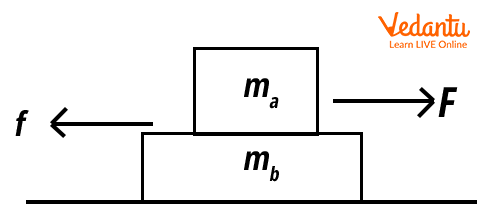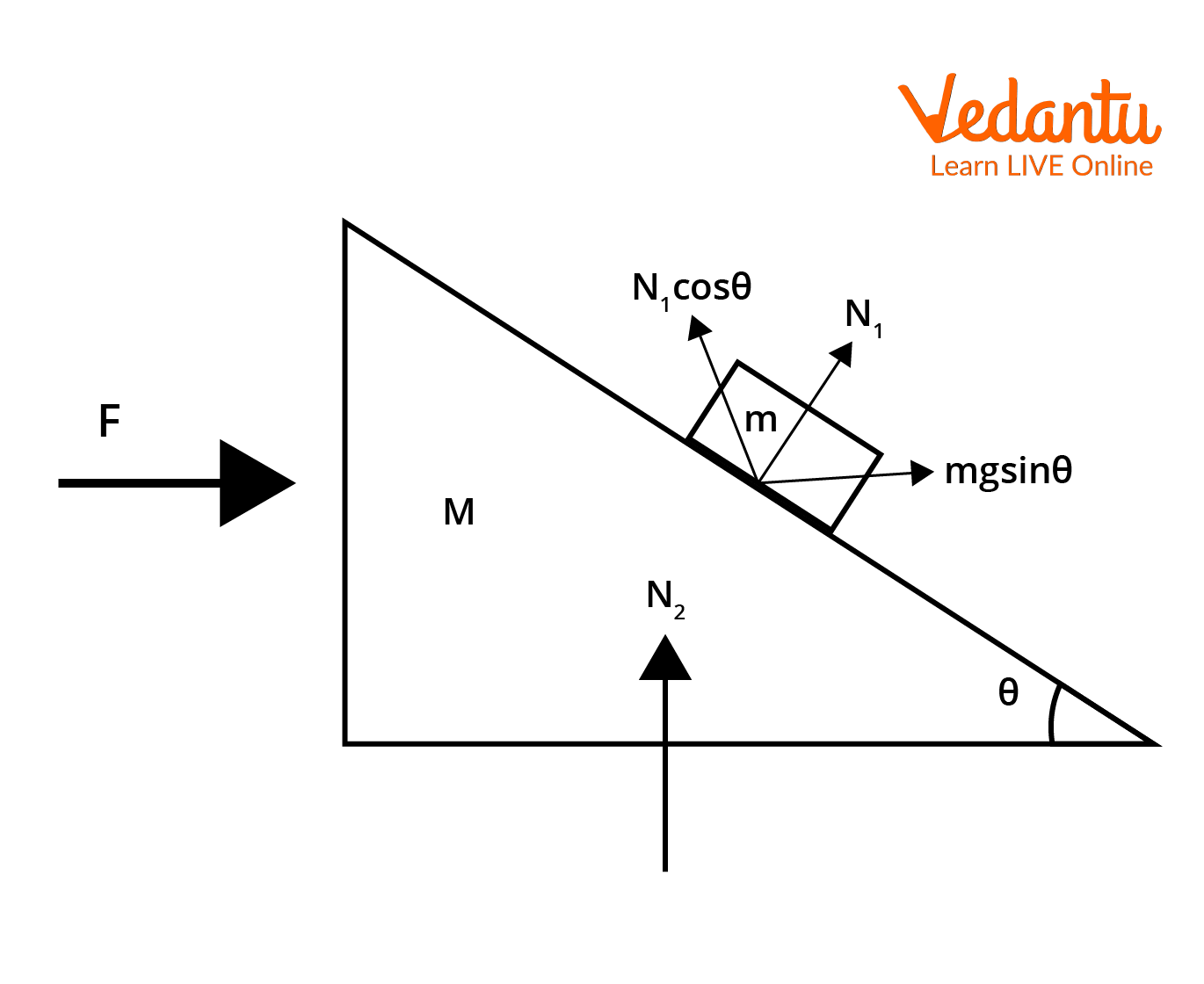Courses
Courses for Kids
Free study material
Free LIVE classes
More

# Block on Block Problems - Friction | JEE Important Topic## Friction Problems: Overview

Last updated date: 18th Mar 2023
Total views: 77.7k
Views today: 1.56k

Mechanics is the branch of science that deals with the forces and their effects on objects which causes motion to it. It involves the analysis of the object’s motion on the application of force and the resisting forces on the object. Mechanics involves various types of principles, including Newton's law of motion, gravity force, and frictional force.

The frictional force is a type of negative force which is used to resist the motion of the body. This concept brought various types of friction problems, ways to find friction force acting on block, block on block problems, block and block friction problems, etc. This article has also illustrated the problems when the force f is applied to the triangular block in step by step manner. In the given article, the block-on-block problems friction involved, friction problems discussed in the upcoming section.

## What is Friction?

Friction is defined as the resisting forces that occurred between the mating surfaces when moving against each other. It is a type of negative force as it acts against the motion of the object. The force of friction always acts against the motion of the object. There are two types of friction which are shown below:

• Sliding Friction

Sliding friction is the resisting force that occurs when two objects slide toward each other.

• Rolling Friction

Rolling friction is the resisting force that occurs when one object starts rolling on the other object.

According to the formula point of view, the frictional force is directly proportional to the normal force on the object. The constant of proportionality is known as the coefficient of friction. The entire formula is shown below:

$f=\mu R$

Here

f = Frictional force, $\mu=$Coefficient friction force and R = Normal force

## Block on Block Friction Problem

The block on block problems friction can be understood as one block kept on the top of the other block and both kept on a frictionless table. Let the mass of the upper block be mu and the mass of the lower block be ml. Now, there can be two cases in case of the block-on-block friction problems which are discussed below:

Case 1: Consider the lower block is pulled in a direction with a force ‘F’. There is no friction acting between the lower block and the table on which it is kept.When a lower object is pulled with a force F

As there is no frictional force existing between the lower block and table, then whenever the lower block gets pulled, the maximum acceleration to which the system of two blocks move together without slipping is evaluated below:

The frictional force acting on the upper block will be $f=\mu mg$. Therefore, the acceleration is produced on the upper block by the frictional force. Hence,

Frictional force = acceleration on upper body

$\mu m_{a} g =m_{a} a$

$a =\mu g$

Therefore, the maximum amount of applied force on which both blocks move together will be

$F=(m_a+m_b)\mu g$

In case, the frictional force exerted between the two blocks and the acceleration is more than the frictional force, then the block will slip with respect to each other. In that case, the acceleration of both the blocks will be different.

The acceleration of the upper block will be $a=\mu_k g$

The acceleration of the lower block will be $a^{\prime}=\dfrac{F-\mu_k m_a g}{m_b}$

Case 2: When the force is applied to the upper block, there will be no friction between the lower block and the horizontal surface.When an upper object is pulled with the force F

In that case, when the force is applied to the upper block, the lower block will automatically be accelerated with the help of frictional force. Then, the maximum acceleration of the system of two blocks will move together without slipping conditions.

$a=\mu_s \dfrac{m_a}{m_b}g$

Here, $\mu_s$ will be the coefficient of static friction between the two blocks. In the context of the above derivation of maximum acceleration, the maximum force which is required to move both the block together will be,

$F=\mu_s\dfrac{m_a}{m_b}g(m_a+m_b)$

## Triangular Block Problem

Suppose there is a block of mass “m” resting on the triangular block of mass “M”. When the force f is applied on the triangular block.  It is shown below:Free body diagram of Triangular with a block resting over it

The analysis of forces that were applied to the block resting on the triangular block will be shown below:

As the triangular block is resting initially on the ground, then the weight of the triangular block (acting downward) is balanced by the normal force “N2” (acting upward) to the bottom surface of the triangular block.

As the force “F” is applied on the triangular block, then the resultant acceleration of the combined system of triangular and block resting on it will be:

\begin{align} &F=(M+m) a \\ &a=\dfrac{F}{(M+m)} \end{align}

Due to the applied force “F”, the forces balanced by block “m” will be solved as,

According to the FBD of small block “m”, the vertical forces are balanced as;

\begin{align} &N_{1} \cos \theta=m g \\ &N_{1}=\dfrac{m g}{\cos \theta} \end{align}

The horizontal forces will be balanced as;

\begin{align} &N_{1} \sin \theta=m a \\ &\dfrac{m g}{\cos \theta} \times \sin \theta=m \times\left(\dfrac{F}{M+m}\right) \\ &m g \tan \theta=m \times\left(\dfrac{F}{M+m}\right) \\ &g \tan \theta=\left(\dfrac{F}{M+m}\right) \\ &g \tan \theta(M+m)=F \end{align}

On rearranging, the net value of force will be found as;

$F=(M+m) g \tan \theta$

## Application of Friction

There are various application of friction in our day to day activities some of them are mentioned below:

• A man can walk on the ground with the help of friction acting between the foot and the ground, absence of friction causes slipping.

• Warmth felt due to rubbing of hands is due to friction acting between our palms while rubbing.

• Fire can be ignited with the help of friction by striking the edges of the stones against each other near the fossil fuels like wood.

• Brakes in the vehicle work completely on the concept of friction.

## Conclusion

This article concluded with the brief concept of friction along with the analysis of block-over-block friction problems. It covers the systematic approach to that problem of the system where the force f is applied on the triangular block with a small block resting on it. It has also found the friction force acting on block, ways to find friction force acting on the block, and discussed the complex level of the block over block problems too. It also covered the concept of mechanics and ended with the application of friction force.

## FAQs on Block on Block Problems - Friction | JEE Important Topic

1. How does the weight of a body affect frictional force acting on it  ?

Friction is the basically the backward force acting on body which resist its forward motion. Frictional force acting on a body can be calculated by the product of weight of the body with coefficient of friction and acceleration due to gravity. Therefore the frictional force on a body is directly proportional to the weight of the body and  any change in  the weight of the body will directly affect the frictional force. For example if the weight of a body is increased four times then the frictional force acting on it will also increase by four times.

2. What is the weightage of friction in the JEE examination?

The weightage of the friction which comes in the section of Newton’s law of motion carries 4 per cent in the jee examination. The expected number of questions in the jee mains examination will be 4-5. This section consists of different varieties of numerical problems relation, friction, block-related problems, rolling friction, triangular block problem, etc. Such topics involve practice and proper understanding of the concept for the preparation for JEE exams.  Hence, this topic is very important for the JEE examination.